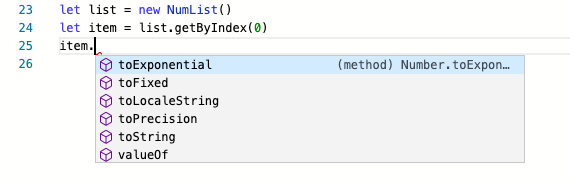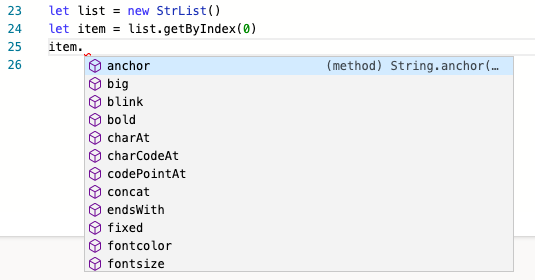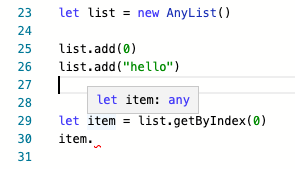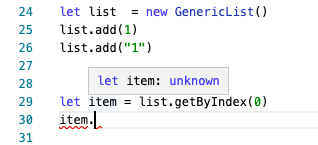## Monday, January 11, 2021

### Introduction to Generics in TypeScript

Generics provides a mechanism for writing code that is flexible enough to apply to a broad range of types but not too flexible that type safety is lost.

What exactly does the above statement mean?

To demonstrate what it means, we will define a class and a function that makes use of Generics. The generic class will be an implementation of a List, which only allows adding and retrieving an item and the generic function will be the identity function

Both the List data structure and the Identity function are good examples because they are straightforward enough to allow for easy demonstration of the Generics concepts.

The idea is to have a List implementation that can hold values of any type. How can such a List be implemented?

If the values will be of number type such an implementation would look like this:

``````class NumList {
private listItems: number[] = []

for (let i of this.listItems) {
if (i == item) {
return;
}
}
this.listItems.push(item)
}

getByIndex(index: number) {
return this.listItems[index];
}

getContent() {
return this.listItems
}
}
``````

And usage would look like this:We see that when we get an item from the list, we have IntelliSense because the compiler knows we are dealing with a variable of type number.

If the values stored in the list will be of type string, such an implementation might look like this:
``````class StrList {

private listItems: string[] = []

for (let i of this.listItems) {
if (i == item) {
return;
}
}
this.listItems.push(item)
}

getByIndex(index: string) {
return this.listItems[index];
}

getContent() {
return this.listItems
}
}``````

And usage would look like this:We also see that when we get an item from the list, we have IntelliSense because the compiler knows we are dealing with a variable of type string.

But there is a problem here. This approach is not scalable. It is not hard to see that we would need an infinite number of implementations for the infinite amount of types we can have.

The question is, is there a way to write an implementation of List that applies to a broad range of types, in this case, any type, without having to repeat implementations?

Well, if we want an implementation that works on all types, then a stab at this will involve using the type any for the arguments. i.e:

``````class AnyList {
private listItems: any[] = []

for (let i of this.listItems) {
if (i == item) {
return;
}
}
this.listItems.push(item)
}

getByIndex(index: any) {
return this.listItems[index];
}

getContent() {
return this.listItems
}
}
``````

This works, the only problem is, we lose type safety. Once we add an item, all type information is lost. As can be seen below. For example, we no longer have IntelliSense:And as shown above, It is also possible to add items of any type, even when the list should contain items of a particular type.

This is where Generics come in. It allows for writing code that is flexible enough to apply to a broad range of types but not too broad that type safety is lost.

An implementation of List using Generics could look like this:

``````class GenericList <T> {
private listItems: T[] = []

for (let i of this.listItems) {
if (i == item) {
return;
}
}
this.listItems.push(item)
}

getByIndex(index: any) {
return this.listItems[index];
}

getContent() {
return this.listItems
}
}``````

Now let’s try to use it. A first attempt looks like this:What gives! It seems we did not achieve our aim? We still don’t have that type safety we seek! It is still possible to add values of random types and we still don't have IntelliSense! Where did things go wrong?

The problem is that we did not specify a type for T. And because of this, the typescript compiler assumes a type of List<unknown>. To fix the problem, we have to specifically construct the type that we need.

And bear in mind, the general theme of advanced types in TypeScript is the ability to manipulate and construct types in a more sophisticated manner based on other types. In this case, we want to construct a particular type of List based on the type of value that will be contained in the List.

Hence we can see List<T> as the mechanism that allows us to construct types. This is also referred to as type constructor, a type-level function that allows us to construct types from another type. So in the case where we want the List to contain values of the number type, we can construct the type as follows:

``````let list : List<number>  = new List()
// Argument of type 'string' is not assignable
// to parameter of type 'number'.
``````

And in the case we want to construct List of string we have:

``````let list : List<string>  = new List()
// Argument of type 'number' is not assignable
// to parameter of type 'string'
``````

Using generics, List<number> or List<string> is constructed from type number,  string etc. This type of construction can take whatever type we supply as input and not just string and number. It will also work for custom types we define:

``````type Person = {
name: string,
age: number,
}

let list  = new GenericList<Person>()
``````

Note that TypeScript can infer the type if we pass a value to the constructor. For example, if we change the implementation of List to:

``````class GenericList<T> {
private listItems: T[] = []

// added constructor that allows type inference
constructor(item: T) {
this.listItems.push(item);
}

for (let i of this.listItems) {
if (i == item) {
return;
}
}
this.listItems.push(item)
}

getByIndex(index: any) {
return this.listItems[index];
}

getContent() {
return this.listItems
}
}``````
Usage:
``````let list  = new GenericList("1")
// Argument of type 'number' is not assignable
// to parameter of type 'string'.
``````

Because we passed a value of type string into the constructor, we do not have to explicitly annotate the variable list as List<string>. The compiler is able to figure this out and enforce the type safety by preventing adding of a value of type number.

Next, we take a look at the identity function and how we can apply the mechanism of generics in its implementation.

We will be adding type annotations to the code examples using Call signature and defining the identity function using function declaration, as this makes the generic annotations more obvious. If you have no idea what Call signature or function declaration is, then I suggest first take a break and read How to apply type annotations to functions in TypeScript.

So let's see how such an identity function may be implemented for the string type.

``````interface IdString {
(item: string):string
}

function identity(input: string) {
return input;
}
``````

And usage will look like:

``````let result = identity("hello")
console.log(result) // prints hello
``````

For a number type, it will look like this:

``````interface IdNumber {
(item: number):number
}

function identity(input: number) {
return input;
}
``````

And usage will look like:

``````let result = identity(13)
console.log(result) // prints result
``````

Again, It is not hard to see the problem here. We would need an infinite number of implementations to support an infinite amount of types we can have.

The question is, is there a way to write an implementation of identity such that it applies to a broad range of types, in this case, any type, without having to repeat implementations?

Well if we want a function that works on all types, then a stab at this will involve using the type any for the arguments. i.e:

``````interface IdAny {
(item: any):any
}

function identity(input: any) {
return input;
}
``````

And can be used with a number or a string as follows:

``````console.log(identity(13))
console.log(identity("13"))
``````

This works, the only problem is, we lose type safety. Once the identity function is used, all type information of the output, which stems from the input is lost. We also lose the ability to have IntelliSense.

``let result = identity("hello")``

Even though we know that result will be of type string the compiler can’t know this and our type safety goes out of the window. This is why using any is not a recommended solution to this problem.

This is where Generics come in. As stated before it allows for writing code that is flexible enough to apply to a broad range of types but not too flexible that type safety is lost.

So we want to have an implementation of identity that works with any type, but still, preserve type information and type safety. Such an implementation will look like this:

``````function identity<T>(input: T) {
return input;
}
``````

Then usage with a will look like as follows:

``````console.log(identity(13))
console.log(identity("hello world"))``````

Again, bear in mind, the general theme of advanced types in TypeScript is the ability to manipulate and construct types in a more sophisticated manner using other types. In this case, we want to construct a particular type for function identity based on the type of value that will be passed in as arguments.

This is exactly what we have done, the type of  identity is now based on whatever type the input is. To make this obvious we can assign the identity function to a variable first, and add explicit type annotation using call signature. That is:

``````interface Id<T> {
(input: T): T
}

function identity<T>(input: T) {
return input;
}
``````

This allows the ability to construct the generic type for the function based on the input argument.

So in the case where we want the identity function to be applied to the argument of number type, we can construct the type as follows:

``````let idNum: Id<number> = identity
console.log(idNum(13))
``````

While for identity function to be applied to argument of string type, we construct that as follows:

``````let idStr: Id<string> = identity
console.log(idStr("Hello"))
``````

Using generics, we were able to construct the type Id<number> or Id<string> based on the type of the input being number and string respectively. This mechanism is not limited to type number or string and shows how Generics provides a mechanism to construct types based on other types. A mechanism that allows for writing generic code without losing type-safety.

This post is part of a series. See Introduction to Advance Types in TypeScript for more posts in the series.

I am writing a book: TypeScript Beyond The Basics. Sign up here to be notified when it is ready.